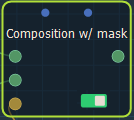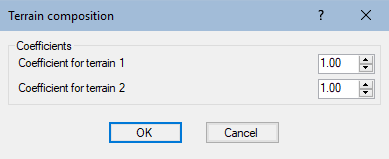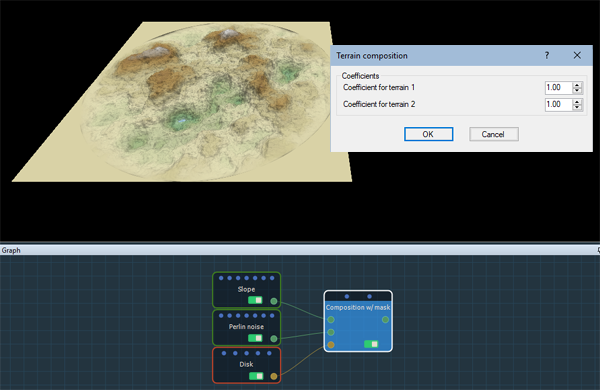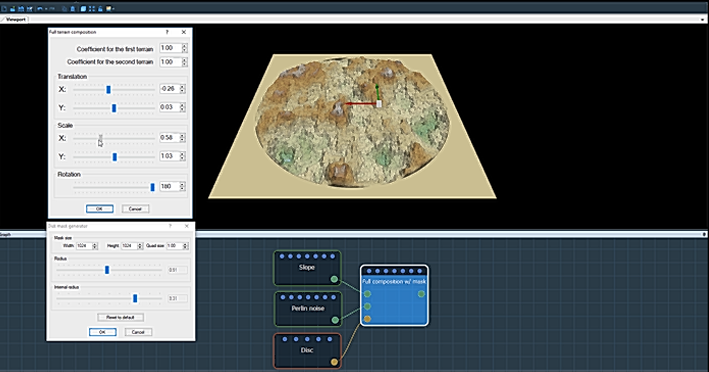This node creates a linear composition between two terrains at locations defined by a mask.

To add a node, right click in the Graph Editor and select Create Node  > Terrain Composition > Composition using mask.Double click on the node to open its parameters:Setting the coefficients

The principle of the Composition using masks node is as follows:

At each vertex:

• If the mask value is equal to 1 (white color), the height of the output terrain is equal to the first input terrain multiplied by the first coefficient, plus the height of the second input terrain multiplied by the second coefficient.
• If the value of the mask is equal to 0 (black color), the height of the output terrain is equal to that of the first input terrain multiplied by the first coefficient. The second input terrain is therefore ignored for this vertex.
• If the mask value is between 0 and 1, the height of the output terrain is equal to the height of the input terrain multiplied by the first coefficient, the height of the second input terrain multiplied by the value of the mask, then multiplied by the second coefficient.

Both input terrains and the input mask can have different sizes and resolutions. The size and resolution of the output terrain are determined by those of the first terrain and the second terrain and the mask.

Set the coefficients for the first and second terrain in the parameters dialog and see how the terrain changes.

• Coefficient for the first terrain: The coefficient by which the height of the first input terrain is multiplied.
• Coefficient for the second terrain: The coefficient by which the height of the second input terrain is multiplied.- When both coefficients are equal to 1, the result is identical to that produced by the Sum node.
- When the first coefficient is equal to 1 and the second is equal to -1, the result is identical to that produced by the Difference node.
- When both coefficients are equal to 0.5, the result is identical to that produced by the Average node.

Video tutorial

Watch our Composition using mask nodes video tutorial, which explains how both the Composition using mask node and the Full composition using mask node work.Parameters

Parameter Use
Coefficient for the first terrain The coefficient by which the height of the first input terrain is multiplied
Coefficient for the second terrain The coefficient by which the height of the second input terrain is multiplied